# Double Clothesline – Solving Equations

I have always taught solving 2-step linear equations by starting with a balance scale. Having students whittle their way down to see how many marbles were in each bag was always a win for me…..in most cases.I valued this approach. It’s easy to visualize and it strengthens the “whatever you do to one side of an equation you do to the other” mantra we tell students when solving . However, I’ve always been left wanting more especially when we introduce solving equations with negative coefficients or even when the solution is a negative value. The balance scale kinda loses it’s effectiveness.

Using algebra tiles help fill this hole. And now…. thanks to Andrew Stadel, double clotheslines.

I was lucky enough to attend Andrew’s NCTM Annual session on Error Analysis this year. In his session he demonstrated how to use a double clothesline to solve equations. I later found this resource on his site. Watch his videos on how to use the clotheslines….they helped me piece this lesson together. Stop now and go and watch Andrew’s video on solving two step equations.

I stared as Andrew did at the NCTM session:

I put 0 on the top line and 0x on the bottom line.I then held up the 3x card and asked where should this go? I asked if it should go on the left or the right of zero. The students overwhelming said it needed to go on the right. “3x is more than x, so it should go more to the right, just like a number line” (Always — Sometimes — Never was going through my head at this moment but i’ll wait to talk about this with the kids until a bit later in the lesson).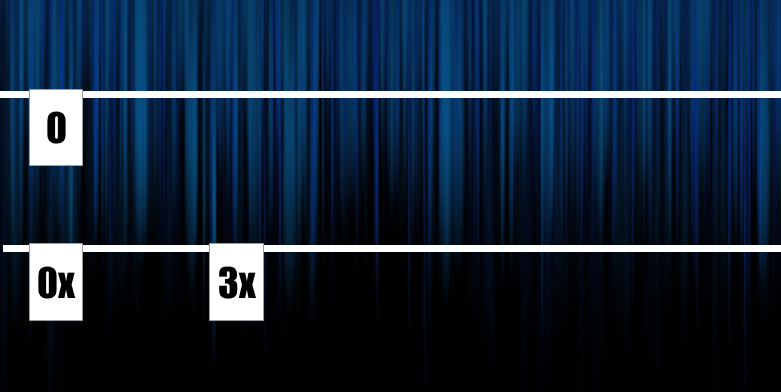I then said “I’m going to place this 15 right above the 3x and that means equivalence. 3x is the same as 15”

Where should 9x go? You could see the some students spacing out where 9x should go. This is what I love about this method. It’s so visual and we’re forced to always think about how terms relate to each other.

I want to know what number should be above 9x. I had them draw the number lines on their desks and let them work on determining the value of 9x.Going around the room there were a few different types of solutions. Some students said, “3 times 3x is 9x, so 3 times 15 is 45”

Some students said, “If 3x is 15 one x is 5, so 9x is 45.” Nice. We ensured the whole class understood both of these types.

Next puzzle: I asked where to place 3x + 4…then assigned it the value of 16.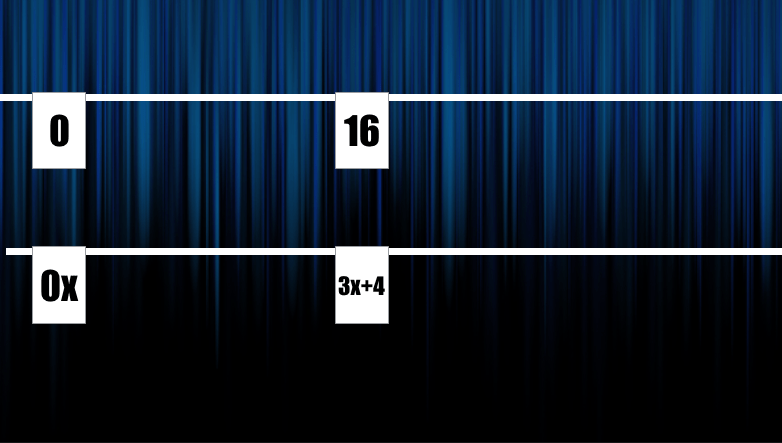Where should 3x be placed? It was easy to see that 3x is less than 3x + 4 so it should go to the left. Now for the amazing moment! What should be the number above?from the class an overwhelmingly 12 was shouted. So now what must be the value of x?Student: “The dividing is the easy part” We spent a few minutes here talking about why dividing 12 by 3 here makes sense.

Next Puzzle:Where should the 5x go? At first some students had some difficulty deciding if it should go to the left or right of 5x – 2.Once we settled to the right. They jumped to finishing it off to determine x.

Next Puzzle:Where 3x should go was a discussion. We all agreed it should be 14 down…..and where would that be? This is where the clothesline (number line) feels superior and the balance scale visual falls short. We can use the bi direction of the number line to continue working with negative values.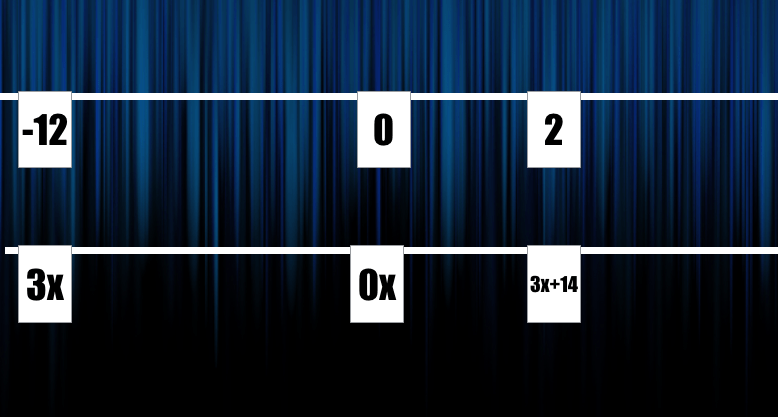What was awesome during this class was this wasn’t a big deal….the number lines seems natural!!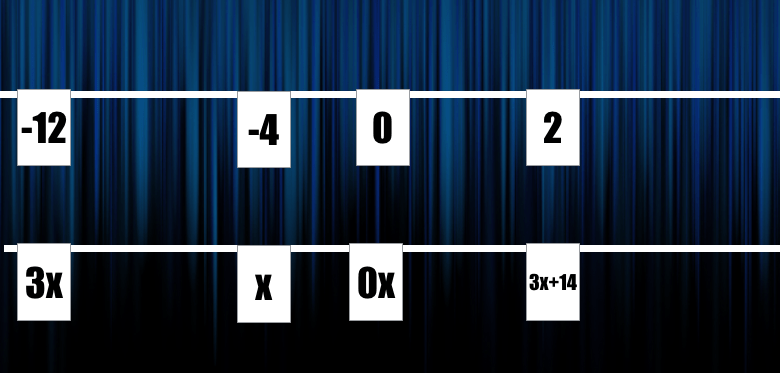Also watch Andrew’s example with negative coefficients.

I had students practice solving a variety of equations by drawing the cards on their handout.They finally demonstrated their understanding by creating their own equation where x had to equal 4. They put their creations up around the room for the group to solve.I feel that the number line (clothesline) method builds a lot of great number sense. We get to reinforce our inverse operations as we build from conceptual understanding to abstract. Students’ strengthen their understanding of algebraic expressions and how those expressions relate to others.

I’m now going to investigate how to to demonstrate solving multi-step equations…. 3x + 5 = 2x + 7 using the clothesline. I’m thinking this might be a difficult task. Any ideas????

[UPDATE] – Solving equations with expression on both sides.

Since this lesson my class used the double number line to solve equations like 4x + 10 = 6x + 2. It was great to keep some continuity here while we solved harder equations.

We placed each side of the equation on separate clotheslines just like before.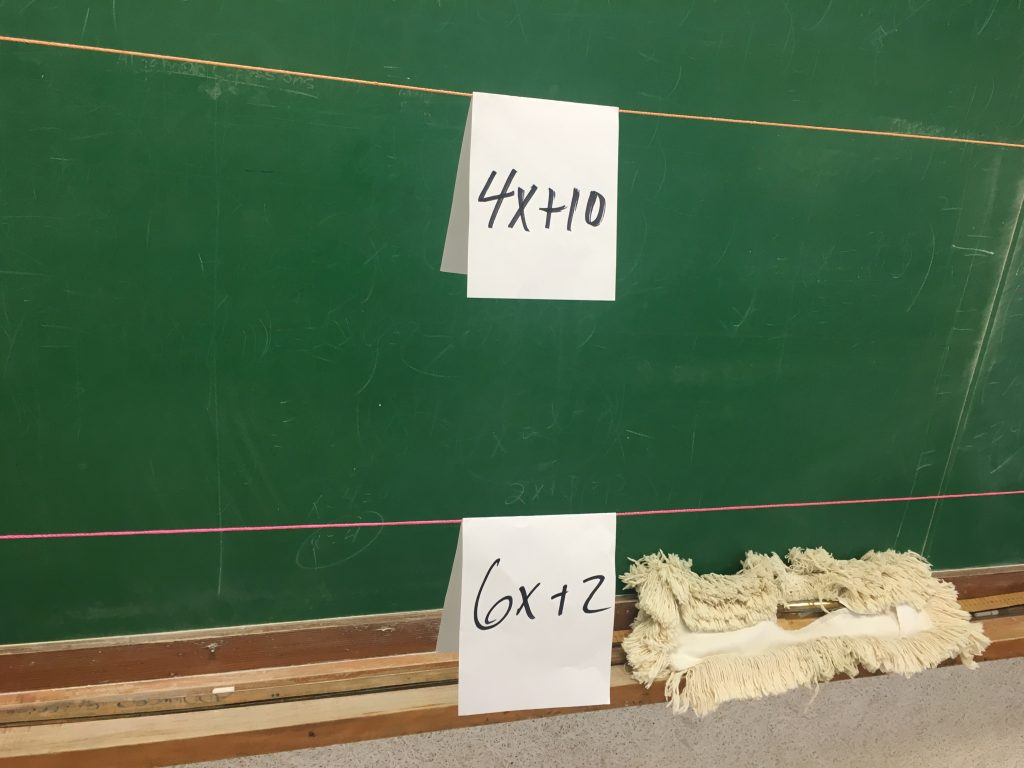We didn’t want to re-invent a new strategy….we were great at solving equations when one line was used for numbers and the other for expressions…..so we wanted that. How can we get one line to be just numbers and one to have the expression? We subtracted 4x from both lines.  Which left us exactly where we were last class!!and then we subtracted 2 from both to isolate the “x-term”Finally dividing by 2Boom!

This will be our method too to solve a system of equations that are both in terms of y.

More clothesline:

# Slope & Clothesline

I’ve loved the idea of using a clothesline in math class. I first read about the strategy from Chris Shore and Andrew Stadel and have been looking for ways to work it into my classroom. Calculating the slope of a line from a graph was coming up in my grade ten 2P course and I thought a clothesline will be a great fit.

We had just finished Fawn’s lesson on steepness with staircases that I found linked from Mary Bourassa’s site. From that lesson my students understood the idea of calculating slope by finding the vertical change and dividing it by the horizontal change, but hadn’t done anything abstract on the coordinate grid.

I wanted my students to:

• Practice calculating slope of a line using two points on a graph.
• Practice calculating slope of a line given two points (no graph shown).
• Compare steepness of lines to other lines using the slope.
• Connect lines that go downward with negative slopes and lines that go upwards with positive slopes.

To start our lesson I asked students if they could calculate the slope of this line:we agreed No. We needed to some measurements! I asked what we could do….a student said “you could give us the grid” Bam! I threw it on there.Enough Now? Still no! We needed the x and y axes.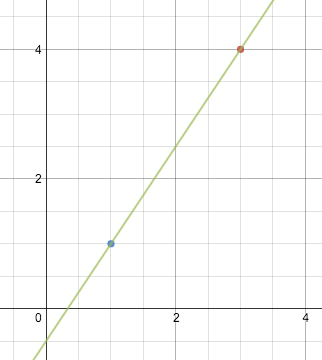As soon as I dropped the axes on…..I could see them all counting and calculating.

We went through calculating the slope of a line like this…and then finally finding the slope a line passing through…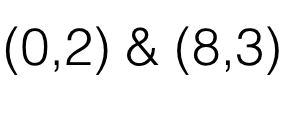It was time to start comparing using a clothesline.

I was originally unsure of how to setup the clothesline for best results as I had never done one. I also wanted to create lines that would give us great results for seeing connections among slope, steepness, and sign value. I enlisted some help from Twitter and recieved some great suggestions

I hung two clotheslines across the room. I placed benchmarks of zero and one on the line. I held up the benchmark of -1 and asked students “where would I place this -1 on the line so it’s right?” They yelled out “more right, more left, LEFT!” until we agreed where it should be.
I had whipped up a set of graphs with lines for students to place on the top clothesline and a set of corresponding ordered pairs for students to place on the bottom clothesline.

Cards looked like: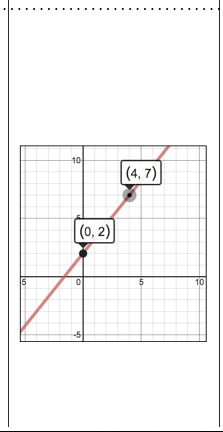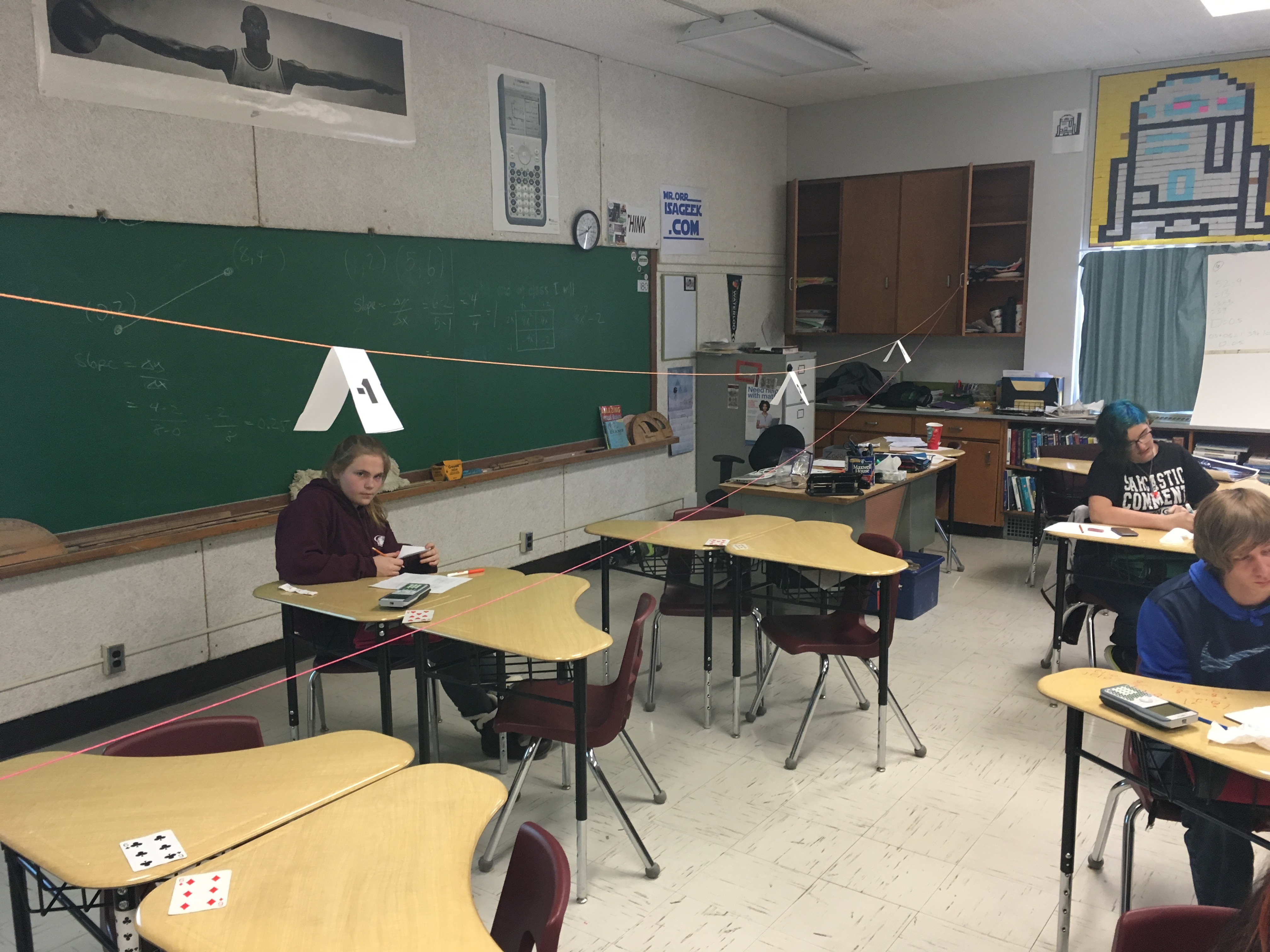I scattered the cards across a table and asked students to choose any card, calculate the slope of the line and then place the card in the right spot on the clothesline.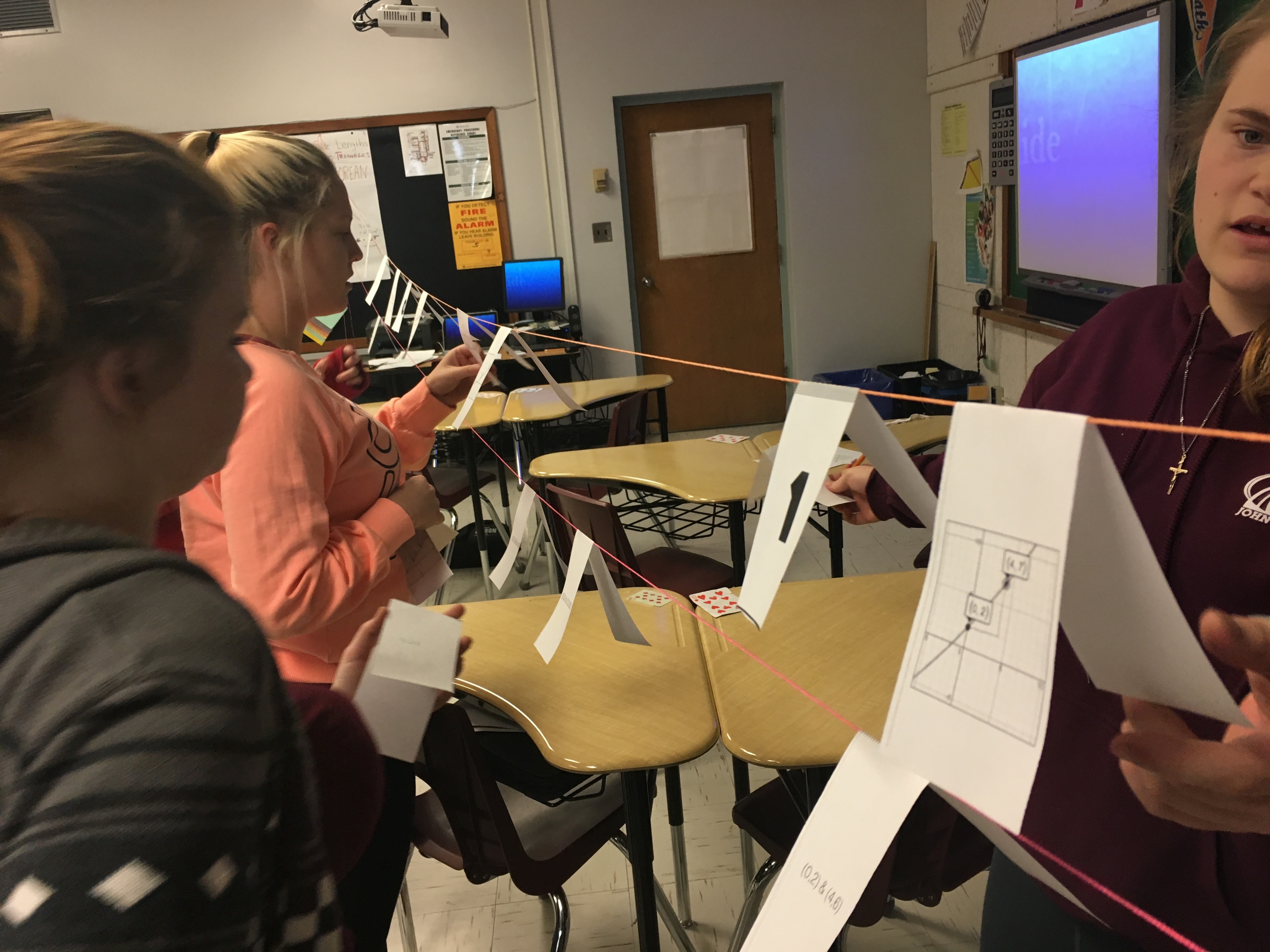The majority of students calculated the slopes fine but were not confident with their answers…and therefore very hesitant on placing the cards on the clothesline. They wanted me to verify their answers before they placed them. They, however did very well determining where to place the cards.

After all cards were placed I noticed a few errors in placement and asked students to go back to the line and check to see if any seemed out of place. We had some great talks on why we knew some were wrong and I heard “All the negative slopes should be on this side” and “that one seems steeper than that one, so it should be here” Once we had placed all the cards we did a gallery walk. I wanted them to see how the steepness changed as we move from negative to positive.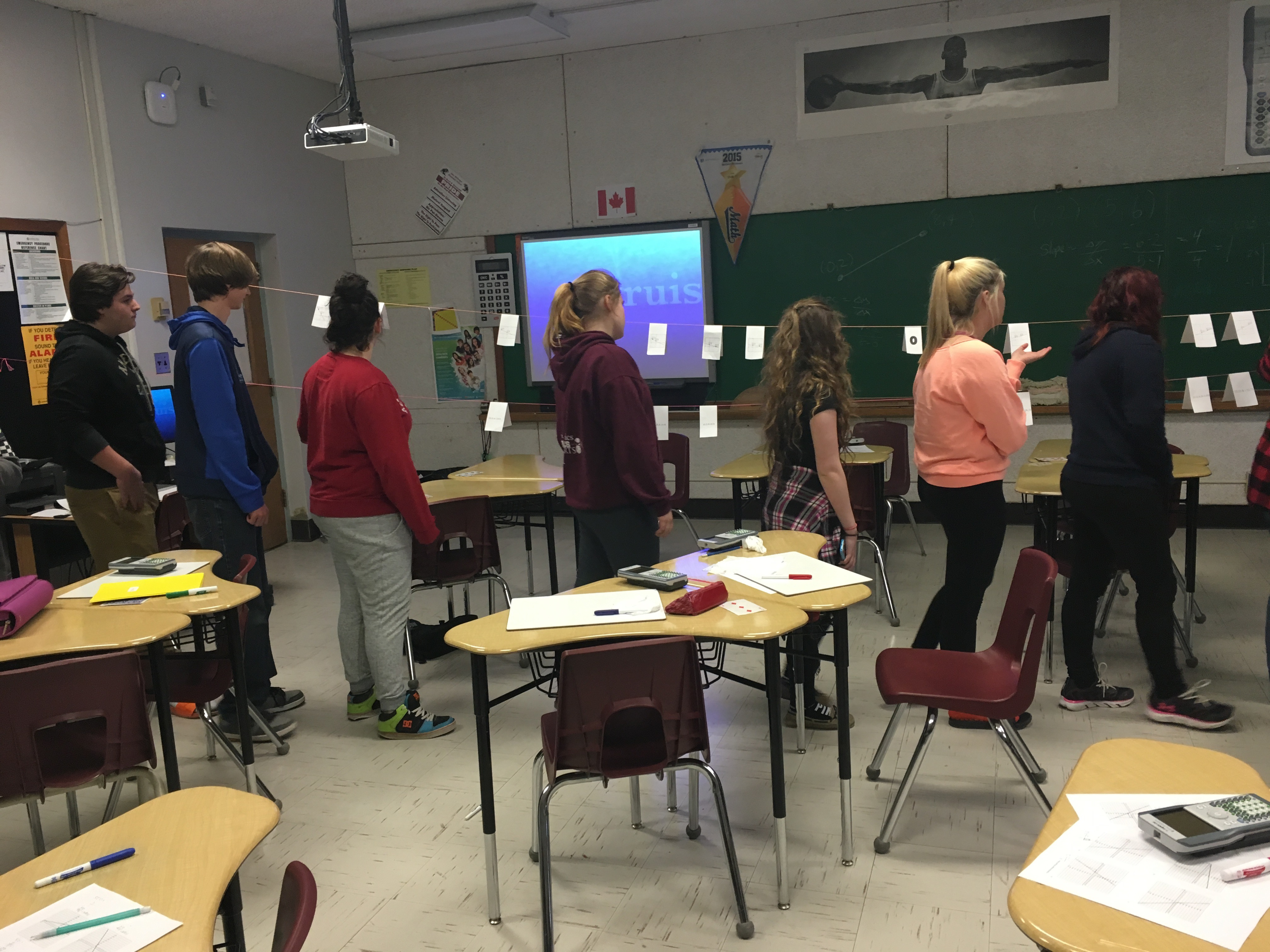This animation shows the gradual change in slope the students would have seen.

We used the patterns to discuss what a line would look like if it had a slope of 0.

There were two lines with a slope of one….I picked them up and we could see talked about parallel lines.

Class finished with us doing two more problems of finding the slope of a line between two points.

I don’t think this lesson was perfect. Could you help me out and provide some suggestions/feedback for me?

Grab the cards: